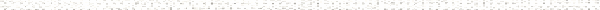An Engineering Statics Outline

 a. Drawing FBDs of a Single SubsystemObjective: Draw the free body diagram for an identified subsystem with engineering connections, representing all interactions with parts external to the subsystem b.Objective: Account properly for forces and couples in equilibrium equations, and deduce their actual senses given their signs found from equilibrium and their assumed sensesObjective: Account properly for pre-modeled known distributed force described as q(x)Objective: Account properly for unknown distributed contact forces, and interpret the results of solution by recognizing physically impossible outcomesObjective: Find the range of a parameter that ensures equilibrium, or the configuration that minimizes or maximizes a forceObjective: Use equilibrium conditions in a qualitative way, without actually solving them, to: make informed assumptions about senses of unknowns, recognize arrangements incapable of being in equilibrium, check the correctness of the senses of forces determined from c.Objective: Choose a solvable subsystem for simple systems (without engineering connections)Objective: Choose a solvable subsystem for systems with engineering connectionsObjective: Choose a solvable subsystem for systems with pulleysObjective: Choose a solvable subsystem by recognizing two-force members when present a. Method of JointsObjective: Solve for forces in trusses using the methods of jointsObjective: Determine forces in bars connected to a single solvable joint b.Objective: Determine internal forces in bars for a given section a. Drawing FBDs of Multiple SubsystemObjective: Represent all interactions with external parts on FBDs of identified subsystems that are parts of a larger system, indicating all their known and unknown attributes, and obeying Newton's 3rd law b.Objective: Solve for all unknowns given a set of subsystems, by imposing successive equilibrium equations each containing one unknown.Objective: Identify sequence of fully solvable subsystems when present, and solve for all unknowns imposing successive equilibrium equations each containing one unknown a. Parallel Force ResolutionObjective: Apply the concepts of two-dimensional statics to the solution of static structures in three dimensionsObjective: Parallel Axis Theorem b. Parallel Force Equilibrium c. Forces in Space d. Concurrent Force Equilibrium e. Non-Concurrent Force Equilibrium a. Plane FrictionObjective: Explain the concepts and theories underlying the nature of friction b. The Laws of FrictionObjective: Define and be able to calculate the coefficients of static and kinetic frictionObjective: Solve a friction problem using analytic, trigonometric, and graphical techniques c. Disc FrictionObjective: Calculate the resisting moment developed by the friction between a surface and a rotating disk (Disc friction) d. Ramp FrictionObjective: Given a specific set of conditions, determine if the state of a body is static, motion impending, moving at constant velocity, or accelerating (Plane and Ramp Friction) e. Wedge FrictionObjective: Calculate the forces developed on a body when multiple surfaces of the body are exposed to friction (Wedge Friction) f. Square Threaded ScrewsObjective: Calculate the torque required to achieve a specific clamping force in a square-threaded screw g. Belt and Wrap FrictionObjective: Determine the tension in a rope or belt system given the geometry of the system and the coefficient of friction a. Moment of Inertia of Plane SectionsObjective: Calculate the area moment of inertia of simple geometric shapesObjective: Definition of the Mass Moment of Inertia b. Moment of Inertia of Composite SectionsObjective: Calculate the area moment of inertia of composite geometric shapes c. Moment of Inertia of Standard SectionsObjective: Determine the area moment of inertia of standard structural sections d. Moment of Inertia of Built-up SectionsObjective: Determine the area moment of inertia of built-up structural sections e. Mohr's Circle for Moment of InertiaObjective: Use Mohr's circle to determine the maximum and minimum area moments of inertia and the rotation of the principle axis about which this occurs for asymmetrical sections